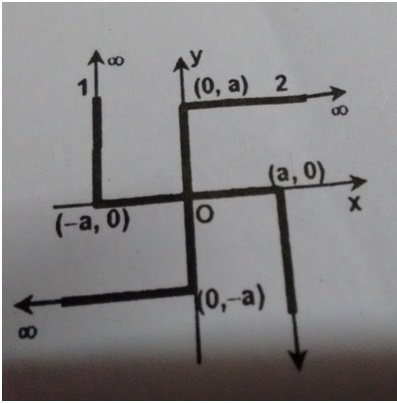Q1) If $cos(x-y)+cos(y-z)+cos(z-x)=\frac{-3}{2}$ and $cosx+cosy+cosz=a$ and $sinx+siny+sinz=b$. Find $a$ and $b$

Q2) Two non-conducting infinite wires have shapes as shown above. The linear charge densities of wire (1) and wire (2) are

$x\quad if\quad x\leq -a~ and~ x\geq a\\ \\ 0\quad if\quad -a

and

$y\quad if\quad y\leq -a~ and~ y\geq a\\ \\ 0\quad if\quad -a

respectively where $(x,y)$ are coordinates of points on the wire. An electron is released from origin, the unit vector along the direction of velocity of the electron just after its release will be in terms of $\hat { i }$ and $\hat { j }$Note by Tanishq Varshney
6 years, 3 months ago

This discussion board is a place to discuss our Daily Challenges and the math and science related to those challenges. Explanations are more than just a solution — they should explain the steps and thinking strategies that you used to obtain the solution. Comments should further the discussion of math and science.

When posting on Brilliant:

• Use the emojis to react to an explanation, whether you're congratulating a job well done , or just really confused .
• Ask specific questions about the challenge or the steps in somebody's explanation. Well-posed questions can add a lot to the discussion, but posting "I don't understand!" doesn't help anyone.
• Try to contribute something new to the discussion, whether it is an extension, generalization or other idea related to the challenge.

MarkdownAppears as
*italics* or _italics_ italics
**bold** or __bold__ bold
- bulleted- list
• bulleted
• list
1. numbered2. list
1. numbered
2. list
Note: you must add a full line of space before and after lists for them to show up correctly
paragraph 1paragraph 2

paragraph 1

paragraph 2

[example link](https://brilliant.org)example link
> This is a quote
This is a quote
    # I indented these lines
# 4 spaces, and now they show
# up as a code block.

print "hello world"
# I indented these lines
# 4 spaces, and now they show
# up as a code block.

print "hello world"
MathAppears as
Remember to wrap math in $$ ... $$ or $ ... $ to ensure proper formatting.
2 \times 3 $2 \times 3$
2^{34} $2^{34}$
a_{i-1} $a_{i-1}$
\frac{2}{3} $\frac{2}{3}$
\sqrt{2} $\sqrt{2}$
\sum_{i=1}^3 $\sum_{i=1}^3$
\sin \theta $\sin \theta$
\boxed{123} $\boxed{123}$

Sort by:

$cosx + cosy + cosz = a$

$\cos^2x + \cos^2y + \cos^2z + 2\cos x\cos y + 2\cos y\cos z + 2 \cos x\cos y = a^2$

Likewise, $\sin^2x + \sin^2y + \sin^2z + 2\sin x\sin y + 2\sin y\sin z + 2 \sin x\sin y = b^2$

Adding, $\cos^2x + \cos^2y + \cos^2z + 2\cos x\cos y + 2\cos y\cos z + 2 \cos x\cos y +$

$\sin^2x + \sin^2y + \sin^2z + 2\sin x\sin y + 2\sin y\sin z + 2 \sin x\sin y = a^2 + b^2$

$\implies 1 + 1 + 1 + 2(\cos x\cos y + \cos y\cos z + \cos x\cos y + \sin x\sin y + \sin y\sin z + \sin x\sin y) = a^2 + b^2$

$\implies 3 + 2(\cos(x-y) + \cos(y-z) + \cos(z-x)) = a^2 + b^2$

$\implies 3 + 2* \frac{-3}{2} = a^2 + b^2$

$0 = a^2 + b^2 \implies a = 0\quad \& \quad b = 0$

- 6 years, 3 months ago

Thanks for this man.

- 6 years, 3 months ago

- 6 years, 3 months ago

The answer to q 1 is very easy: $a=b=0$.

- 6 years, 3 months ago

Ans 2 q2 seems to be zero too.

- 6 years, 3 months ago

can u explain in brief the first part

- 6 years, 3 months ago

for first one The simplest way is to assume all the three angle differences equal, which ofcourse then must be 120 degree, so just consider 1 , w ,w^2 , add em up

- 6 years, 3 months ago